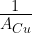Enlightened

# Question 16: NCERT Solutions for 12th Class Physics: Chapter 3-Current Electricity

• 0

Question 16: NCERT Solutions for 12th Class Physics: Chapter 3-Current Electricity

Two wires of equal length, one of aluminium and the other of copper have the same resistance. Which of the two wires is lighter? Hence explain why aluminium wires are preferred for overhead power cables.Share

1. Solution:
Two wires have same length, and resistance. As the specific resistances are unequal, the areas are different. For copper wire, Rcu = pcuThus, the aluminium wire for the same resistance is very light than copper and that is why aluminium wires are preferred for overhead power cables.

Check the complete chapter with solutions.

NCERT Solutions for 12th Class Physics: Chapter 3-Current Electricity

• 0